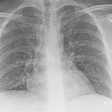# A: Extract the sudoku from the image

## 1: Pre Processing the image

`proc = cv2.GaussianBlur(img.copy(), (9, 9), 0)proc = cv2.adaptiveThreshold(proc, 255, cv2.ADAPTIVE_THRESH_GAUSSIAN_C, cv2.THRESH_BINARY, 11, 2)`
`proc = cv2.bitwise_not(proc, proc)  kernel = np.array([[0., 1., 0.], [1., 1., 1.], [0., 1., 0.]],np.uint8)proc = cv2.dilate(proc, kernel)`

## 2: Find the corners of the largest polygon

`_, contours, h = cv2.findContours(img.copy(), cv2.RETR_EXTERNAL, cv2.CHAIN_APPROX_SIMPLE)contours = sorted(contours, key=cv2.contourArea, reverse=True)polygon = contours`
`bottom_right, _ = max(enumerate([pt + pt for pt in                      polygon]), key=operator.itemgetter(1))top_left, _ = min(enumerate([pt + pt for pt in                  polygon]), key=operator.itemgetter(1))bottom_left, _ = min(enumerate([pt - pt for pt in                     polygon]), key=operator.itemgetter(1))top_right, _ = max(enumerate([pt - pt for pt in                   polygon]), key=operator.itemgetter(1))`
`[polygon[top_left], polygon[top_right], polygon[bottom_right], polygon[bottom_left]]`

## 3: Crop and Warp Image

`top_left, top_right, bottom_right, bottom_left = crop_rect, crop_rect, crop_rect, crop_rectsrc = np.array([top_left, top_right, bottom_right, bottom_left], dtype='float32') side = max([  distance_between(bottom_right, top_right),             distance_between(top_left, bottom_left),            distance_between(bottom_right, bottom_left),               distance_between(top_left, top_right) ])`
`def distance_between(p1, p2):     a = p2 - p1     b = p2 - p1     return np.sqrt((a ** 2) + (b ** 2))`
`dst = np.array([[0, 0], [side - 1, 0], [side - 1, side - 1], [0, side - 1]], dtype='float32')m = cv2.getPerspectiveTransform(src, dst)cv2.warpPerspective(img, m, (int(side), int(side)))`

## 4: Infer grid from the square image

`squares = [] side = img.shape[:1] side = side / 9for j in range(9):    for i in range(9):        p1 = (i * side, j * side)  #Top left corner of a box           p2 = ((i + 1) * side, (j + 1) * side)  #Bottom right corner                 squares.append((p1, p2)) return squares`

## 5: Get each digit

`digits = []img = pre_process_image(img.copy(), skip_dilate=True)for square in squares:    digits.append(extract_digit(img, square, size))`
`def extract_digit(img, rect, size):    digit = cut_from_rect(img, rect)    h, w = digit.shape[:2]    margin = int(np.mean([h, w]) / 2.5)    _, bbox, seed = find_largest_feature(digit, [margin, margin], [w    - margin, h - margin])    digit = cut_from_rect(digit, bbox)         w = bbox - bbox    h = bbox - bbox     if w > 0 and h > 0 and (w * h) > 100 and len(digit) > 0:        return scale_and_centre(digit, size, 4)    else:        return np.zeros((size, size), np.uint8)`

--

--

--

## More from Aakash Jhawar

Software Engineer, Machine Learning

Love podcasts or audiobooks? Learn on the go with our new app.

## Image Inpainting and its evolution: A brief discussion…## Two minutes NLP — Basic taxonomy of Topic Tagging models and elementary use cases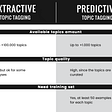## AI - Simulated Annealing Algorithm to solve Magic Square Problem## Impacts of Alluxio Optimization on Kubernetes Deep Learning and Training## Exploring PyTorch Tensor## How to Automate Hyperparameter Optimization## Face Recognition using Transfer Learning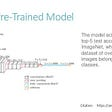## Machine Learning at CSE, IITK — The Culture, Research and Prospects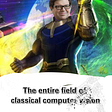## Aakash Jhawar

Software Engineer, Machine Learning

## Feedforward Neural Network (FNN) Implementation from Scratch Using Python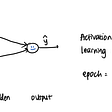## How to Detect Contours in an Image in Python using OpenCV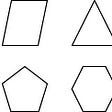## Object recognition and handling overfitting for CIFAR-10 image dataset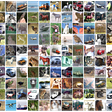## Deep Learning in Medicine: Identifying Pneumonia from a Chest-X-Ray